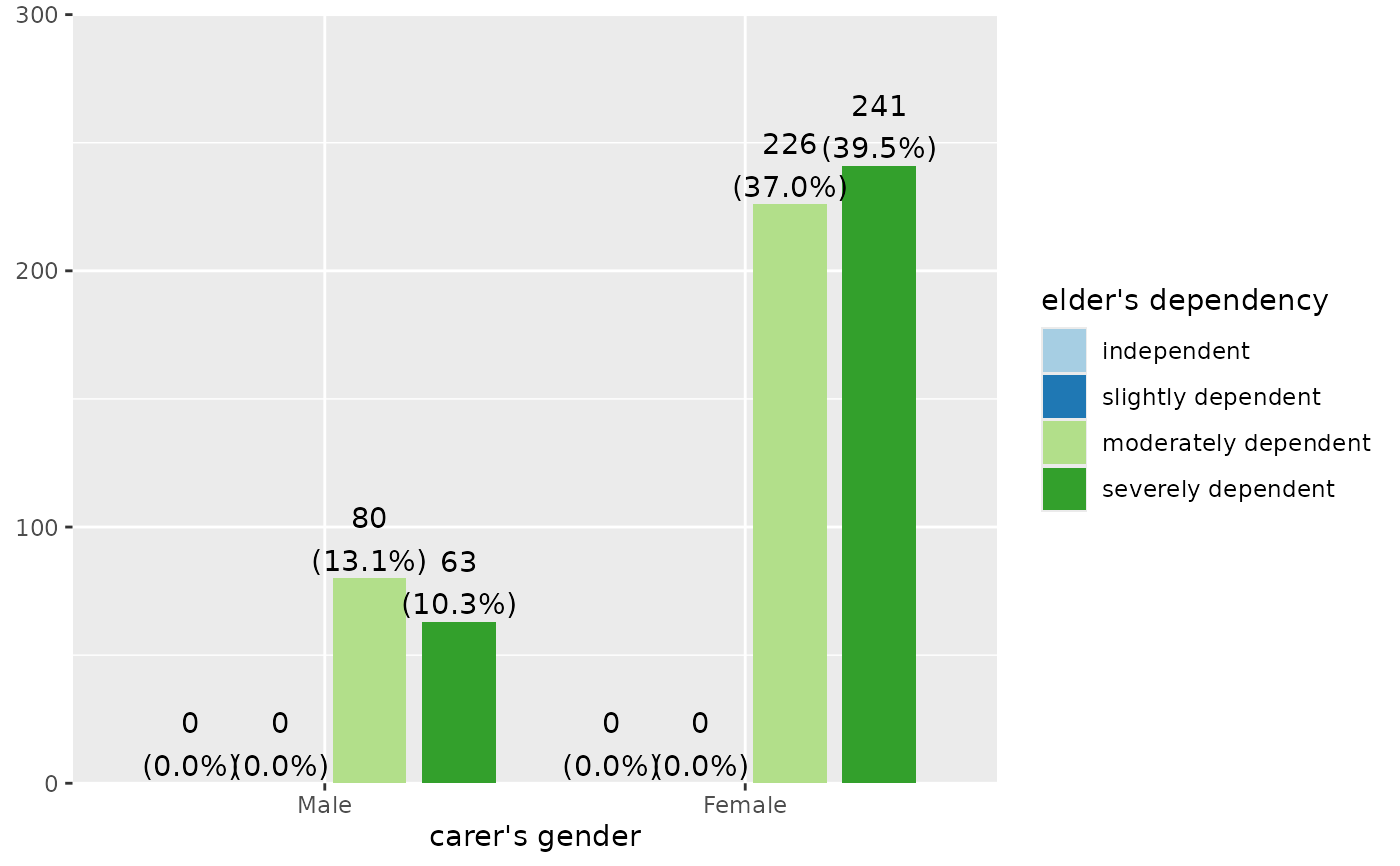Plot grouped or stacked frequencies of variables as bar/dot, box or violin plots, or line plot.

## Usage

plot_grpfrq(
var.cnt,
var.grp,
type = c("bar", "dot", "line", "boxplot", "violin"),
bar.pos = c("dodge", "stack"),
weight.by = NULL,
intr.var = NULL,
title = "",
title.wtd.suffix = NULL,
legend.title = NULL,
axis.titles = NULL,
axis.labels = NULL,
legend.labels = NULL,
intr.var.labels = NULL,
wrap.title = 50,
wrap.labels = 15,
wrap.legend.title = 20,
wrap.legend.labels = 20,
geom.size = NULL,
geom.spacing = 0.15,
geom.colors = "Paired",
show.values = TRUE,
show.n = TRUE,
show.prc = TRUE,
show.axis.values = TRUE,
show.ci = FALSE,
show.grpcnt = FALSE,
show.legend = TRUE,
show.na = FALSE,
show.summary = FALSE,
drop.empty = TRUE,
auto.group = NULL,
ylim = NULL,
grid.breaks = NULL,
expand.grid = FALSE,
inner.box.width = 0.15,
inner.box.dotsize = 3,
smooth.lines = FALSE,
emph.dots = TRUE,
summary.pos = "r",
facet.grid = FALSE,
coord.flip = FALSE,
y.offset = NULL,
vjust = "bottom",
hjust = "center"
)

## Arguments

var.cnt

Vector of counts, for which frequencies or means will be plotted or printed.

var.grp

Factor with the cross-classifying variable, where var.cnt is grouped into the categories represented by var.grp.

type

Specifies the plot type. May be abbreviated.

"bar"

for simple bars (default)

"dot"

for a dot plot

"histogram"

for a histogram (does not apply to grouped frequencies)

"line"

for a line-styled histogram with filled area

"density"

for a density plot (does not apply to grouped frequencies)

"boxplot"

for box plot

"violin"

for violin plots

bar.pos

Indicates whether bars should be positioned side-by-side (default), or stacked (bar.pos = "stack"). May be abbreviated.

weight.by

Vector of weights that will be applied to weight all cases. Must be a vector of same length as the input vector. Default is NULL, so no weights are used.

intr.var

An interaction variable which can be used for box plots. Divides each category indicated by var.grp into the factors of intr.var, so that each category of var.grp is subgrouped into intr.var's categories. Only applies when type = "boxplot" or type = "violin".

title

character vector, used as plot title. Depending on plot type and function, will be set automatically. If title = "", no title is printed. For effect-plots, may also be a character vector of length > 1, to define titles for each sub-plot or facet.

title.wtd.suffix

Suffix (as string) for the title, if weight.by is specified, e.g. title.wtd.suffix=" (weighted)". Default is NULL, so title will not have a suffix when cases are weighted.

legend.title

character vector, used as title for the plot legend.

axis.titles

character vector of length one or two, defining the title(s) for the x-axis and y-axis.

axis.labels

character vector with labels used as axis labels. Optional argument, since in most cases, axis labels are set automatically.

legend.labels

character vector with labels for the guide/legend.

intr.var.labels

a character vector with labels for the x-axis breaks when having interaction variables included. These labels replace the axis.labels. Only applies, when using box or violin plots (i.e. type = "boxplot" or "violin") and intr.var is not NULL.

wrap.title

numeric, determines how many chars of the plot title are displayed in one line and when a line break is inserted.

wrap.labels

numeric, determines how many chars of the value, variable or axis labels are displayed in one line and when a line break is inserted.

wrap.legend.title

numeric, determines how many chars of the legend's title are displayed in one line and when a line break is inserted.

wrap.legend.labels

numeric, determines how many chars of the legend labels are displayed in one line and when a line break is inserted.

geom.size

size resp. width of the geoms (bar width, line thickness or point size, depending on plot type and function). Note that bar and bin widths mostly need smaller values than dot sizes.

geom.spacing

the spacing between geoms (i.e. bar spacing)

geom.colors

user defined color for geoms. See 'Details' in plot_grpfrq.

show.values

Logical, whether values should be plotted or not.

show.n

logical, if TRUE, adds total number of cases for each group or category to the labels.

show.prc

logical, if TRUE (default), percentage values are plotted to each bar If FALSE, percentage values are removed.

show.axis.values

logical, whether category, count or percentage values for the axis should be printed or not.

show.ci

Logical, if TRUE), adds notches to the box plot, which are used to compare groups; if the notches of two boxes do not overlap, medians are considered to be significantly different.

show.grpcnt

logical, if TRUE, the count within each group is added to the category labels (e.g. "Cat 1 (n=87)"). Default value is FALSE.

show.legend

logical, if TRUE, and depending on plot type and function, a legend is added to the plot.

show.na

logical, if TRUE, NA's (missing values) are added to the output.

show.summary

logical, if TRUE (default), a summary with chi-squared statistics (see chisq.test), Cramer's V or Phi-value etc. is shown. If a cell contains expected values lower than five (or lower than 10 if df is 1), the Fisher's exact test (see fisher.test) is computed instead of chi-squared test. If the table's matrix is larger than 2x2, Fisher's exact test with Monte Carlo simulation is computed.

drop.empty

Logical, if TRUE and the variable's values are labeled, values / factor levels with no occurrence in the data are omitted from the output. If FALSE, labeled values that have no observations are still printed in the table (with frequency 0).

auto.group

numeric value, indicating the minimum amount of unique values in the count variable, at which automatic grouping into smaller units is done (see group_var). Default value for auto.group is NULL, i.e. auto-grouping is off. See group_var for examples on grouping.

ylim

numeric vector of length two, defining lower and upper axis limits of the y scale. By default, this argument is set to NULL, i.e. the y-axis fits to the required range of the data.

grid.breaks

numeric; sets the distance between breaks for the axis, i.e. at every grid.breaks'th position a major grid is being printed.

expand.grid

logical, if TRUE, the plot grid is expanded, i.e. there is a small margin between axes and plotting region. Default is FALSE.

inner.box.width

width of the inner box plot that is plotted inside of violin plots. Only applies if type = "violin". Default value is 0.15

inner.box.dotsize

size of mean dot insie a violin or box plot. Applies only when type = "violin" or "boxplot".

smooth.lines

prints a smooth line curve. Only applies, when argument type = "line".

emph.dots

logical, if TRUE, the groups of dots in a dot-plot are highlighted with a shaded rectangle.

summary.pos

position of the model summary which is printed when show.summary is TRUE. Default is "r", i.e. it's printed to the upper right corner. Use "l" for upper left corner.

facet.grid

TRUE to arrange the lay out of of multiple plots in a grid of an integrated single plot. This argument calls facet_wrap or facet_grid to arrange plots. Use plot_grid to plot multiple plot-objects as an arranged grid with grid.arrange.

coord.flip

logical, if TRUE, the x and y axis are swapped.

y.offset

numeric, offset for text labels when their alignment is adjusted to the top/bottom of the geom (see hjust and vjust).

vjust

character vector, indicating the vertical position of value labels. Allowed are same values as for vjust aesthetics from ggplot2: "left", "center", "right", "bottom", "middle", "top" and new options like "inward" and "outward", which align text towards and away from the center of the plot respectively.

hjust

character vector, indicating the horizontal position of value labels. Allowed are same values as for vjust aesthetics from ggplot2: "left", "center", "right", "bottom", "middle", "top" and new options like "inward" and "outward", which align text towards and away from the center of the plot respectively.

A ggplot-object.

## Details

geom.colors may be a character vector of color values in hex-format, valid color value names (see demo("colors") or a name of a color brewer palette. Following options are valid for the geom.colors argument:

• If not specified, a default color brewer palette will be used, which is suitable for the plot style (i.e. diverging for likert scales, qualitative for grouped bars etc.).

• If "gs", a greyscale will be used.

• If "bw", and plot-type is a line-plot, the plot is black/white and uses different line types to distinguish groups (see this package-vignette).

• If geom.colors is any valid color brewer palette name, the related palette will be used. Use RColorBrewer::display.brewer.all() to view all available palette names.

• Else specify own color values or names as vector (e.g. geom.colors = c("#f00000", "#00ff00")).

## Examples

data(efc)
plot_grpfrq(efc$e17age, efc$e16sex, show.values = FALSE)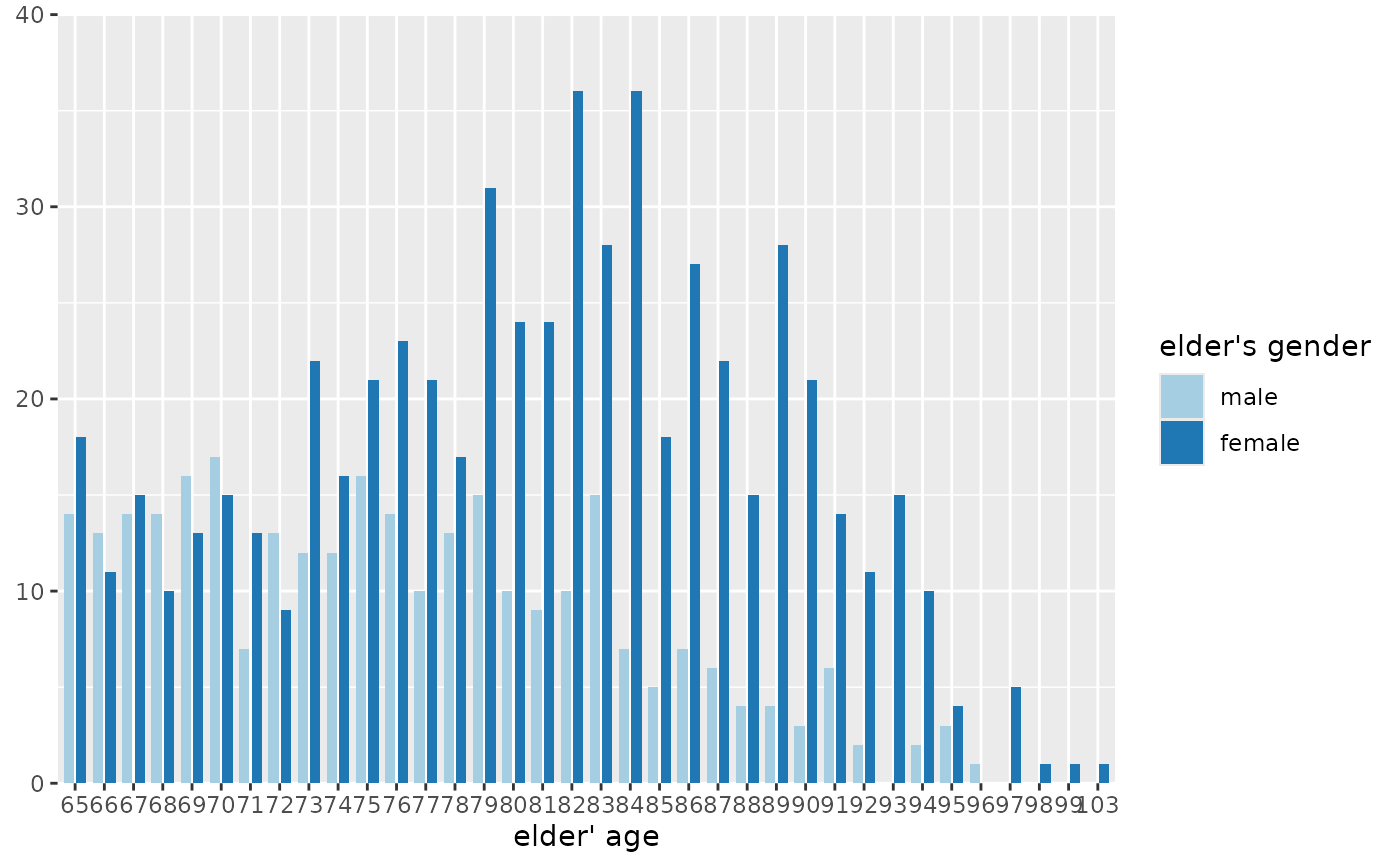# boxplot
plot_grpfrq(efc$e17age, efc$e42dep, type = "box")
#> Warning: Solution may be nonunique
#> Warning: Solution may be nonunique
#> Warning: Solution may be nonunique
#> Warning: Solution may be nonunique
#> Warning: Solution may be nonunique
#> Warning: Solution may be nonunique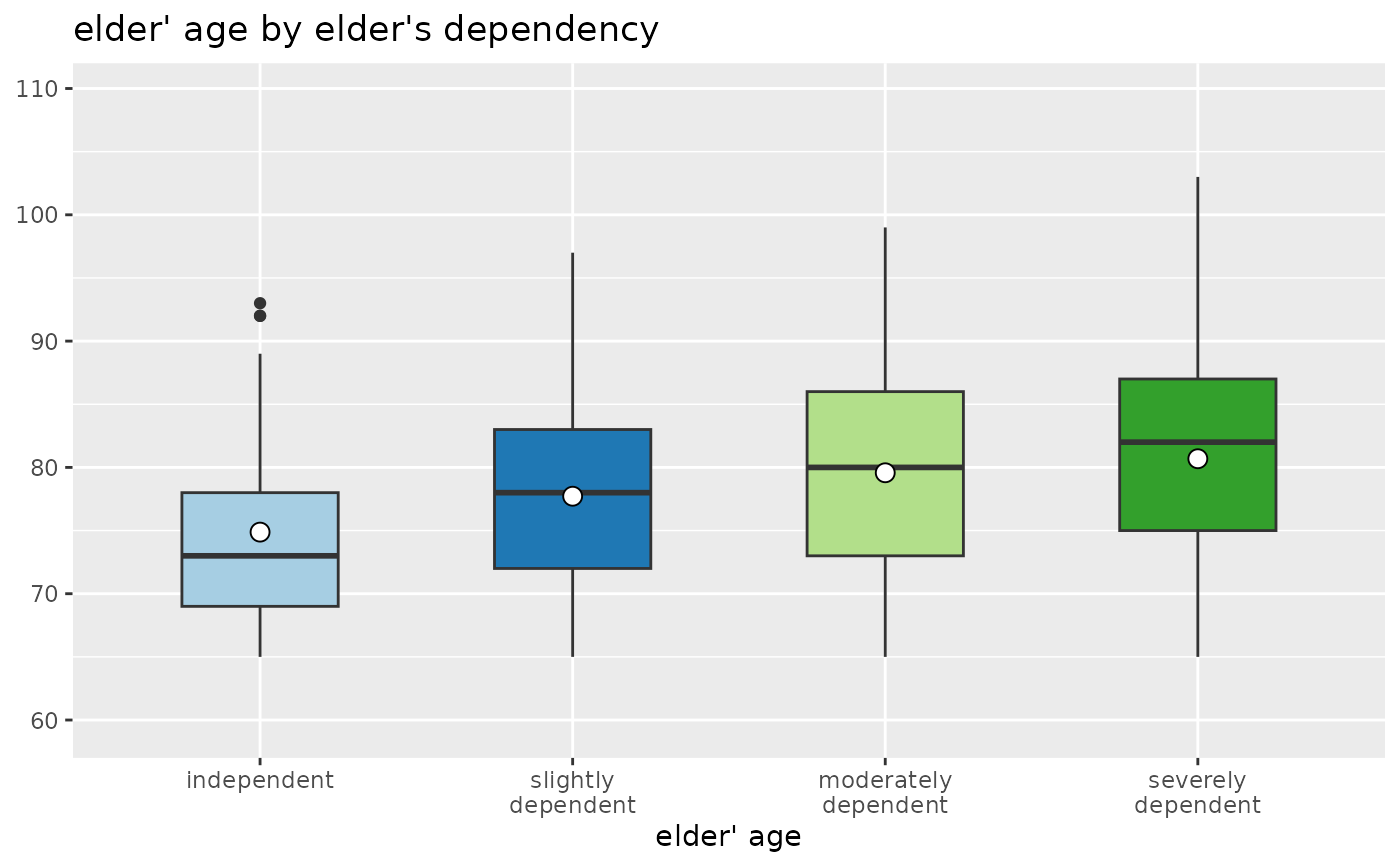# grouped bars
plot_grpfrq(efc$e42dep, efc$e16sex, title = NULL)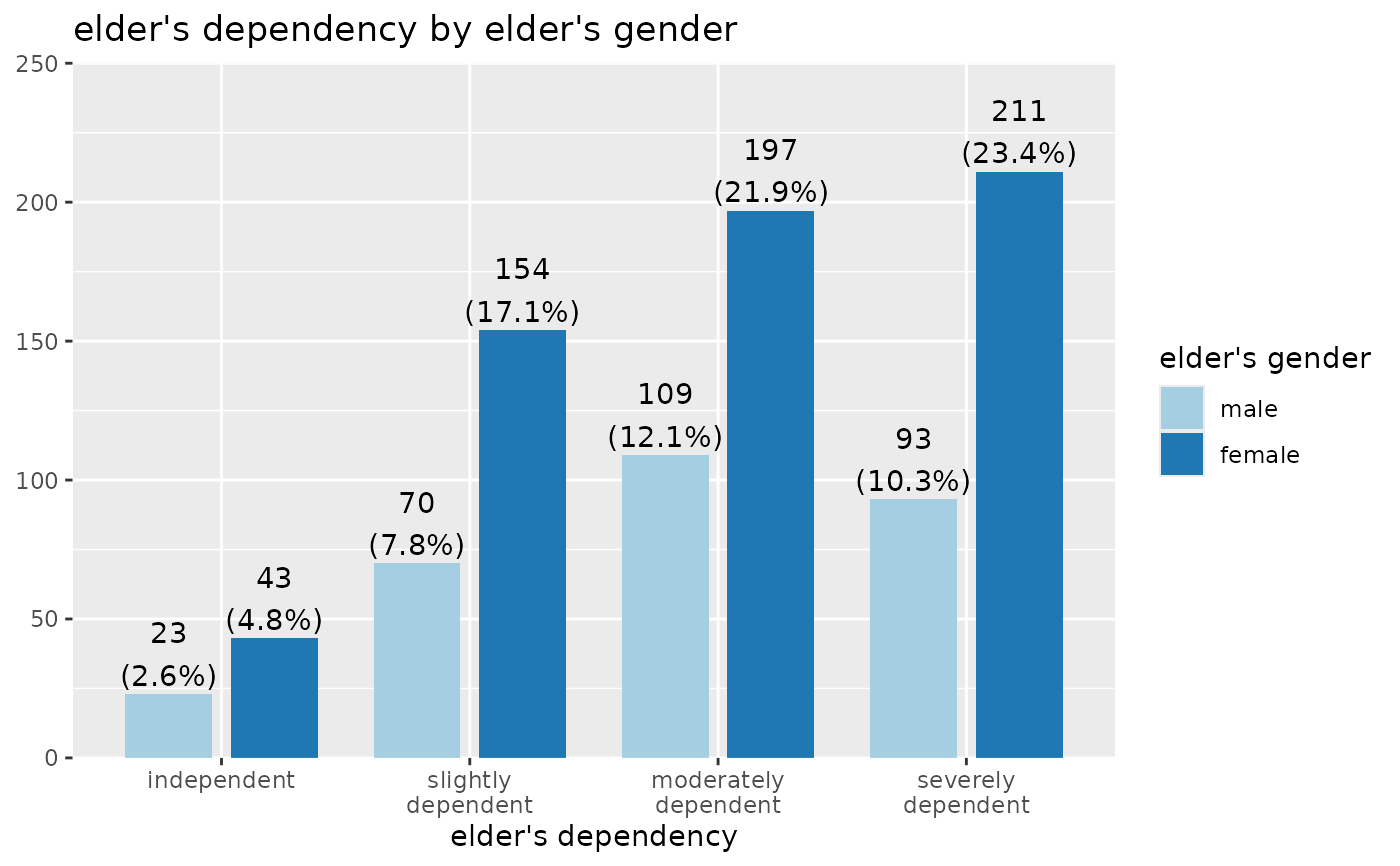# box plots with interaction variable
plot_grpfrq(efc$e17age, efc$e42dep, intr.var = efc$e16sex, type = "box") #> Warning: Solution may be nonunique #> Warning: Solution may be nonunique #> Warning: Solution may be nonunique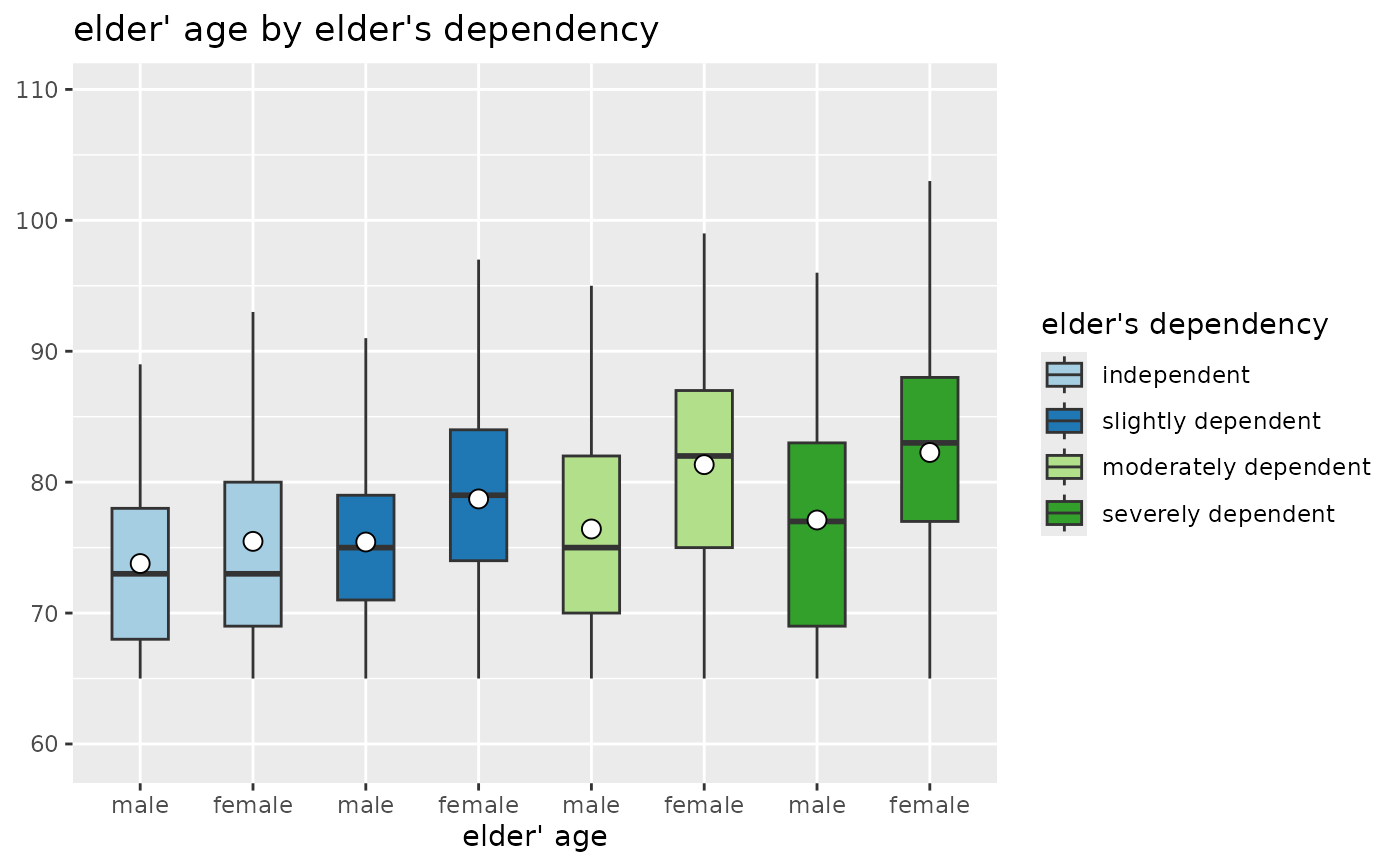# Grouped bar plot plot_grpfrq(efc$neg_c_7, efc$e42dep, show.values = FALSE)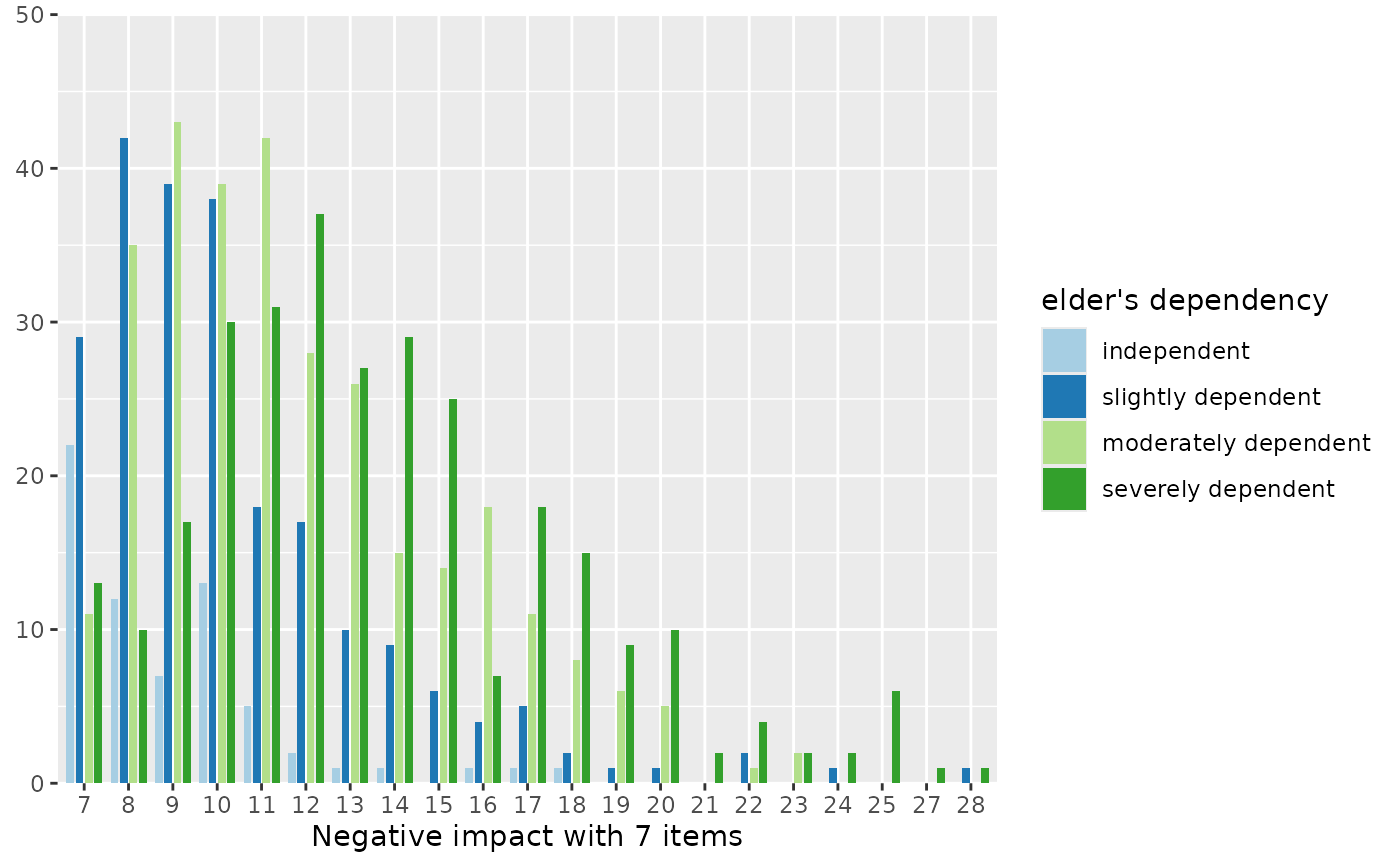# same data as line plot plot_grpfrq(efc$neg_c_7, efc$e42dep, type = "line")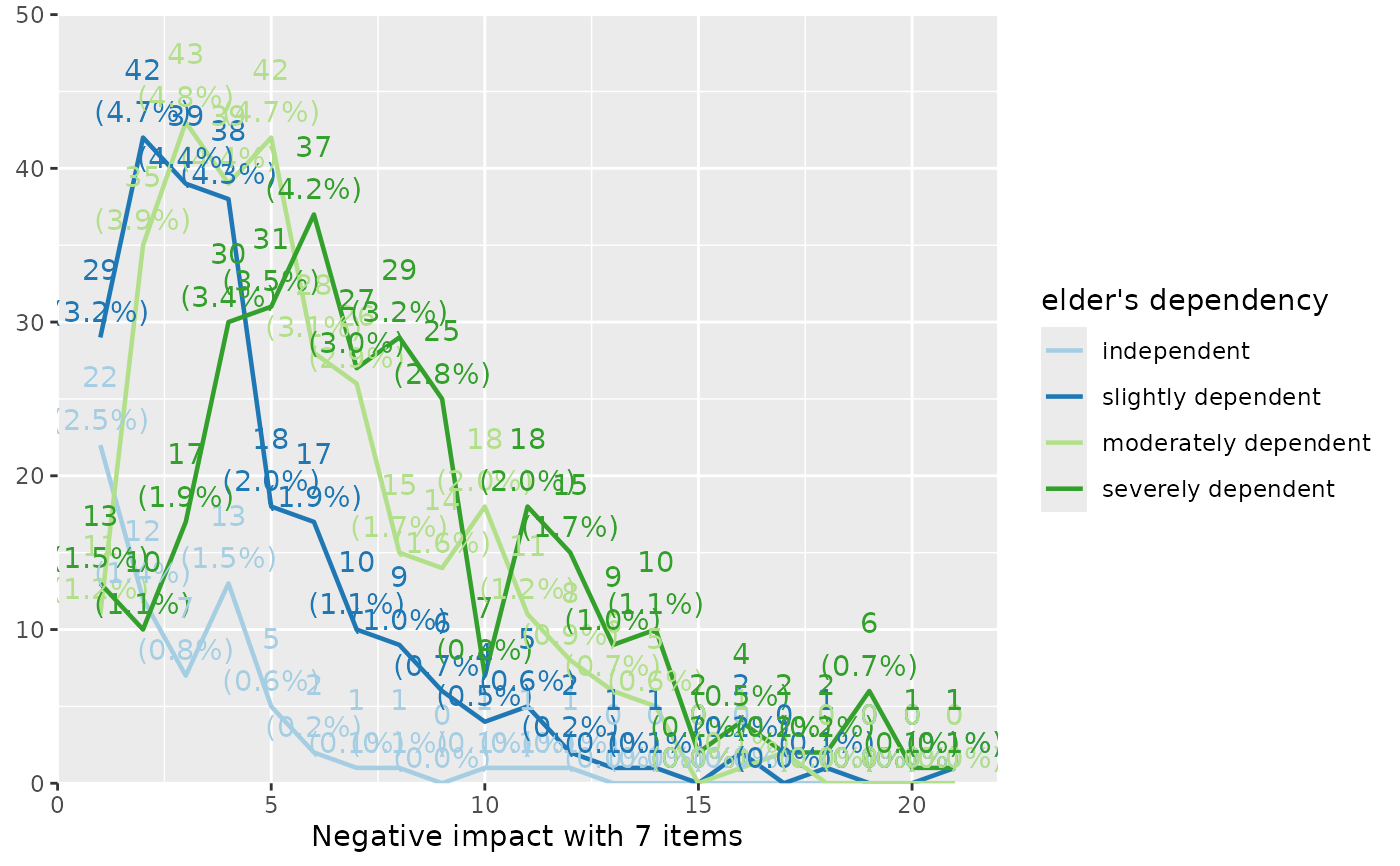# show ony categories where we have data (i.e. drop zero-counts) library(dplyr) efc <- dplyr::filter(efc, e42dep %in% c(3,4)) plot_grpfrq(efc$c161sex, efc$e42dep, drop.empty = TRUE)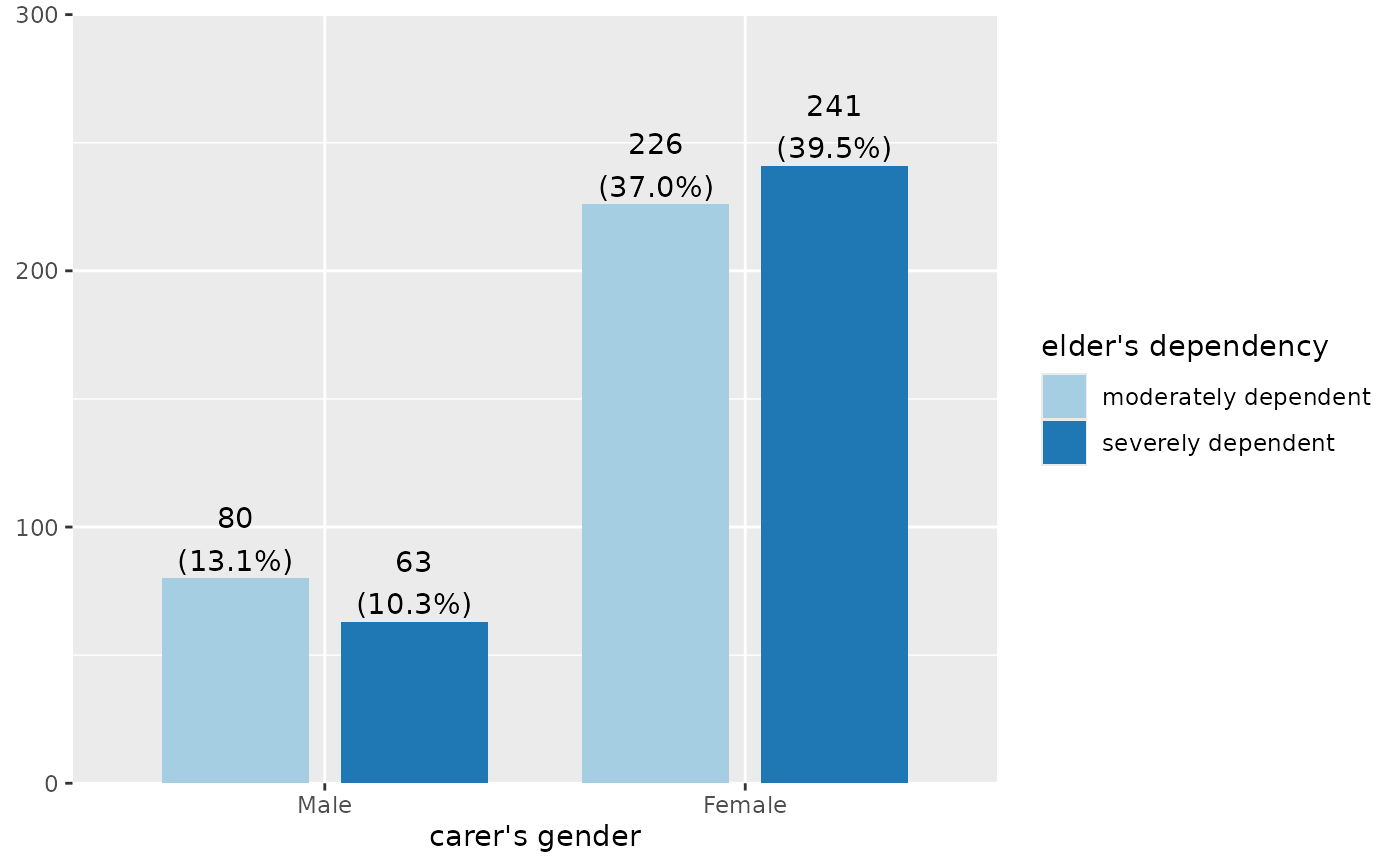# show all categories, even if not in data plot_grpfrq(efc$c161sex, efc\$e42dep, drop.empty = FALSE)